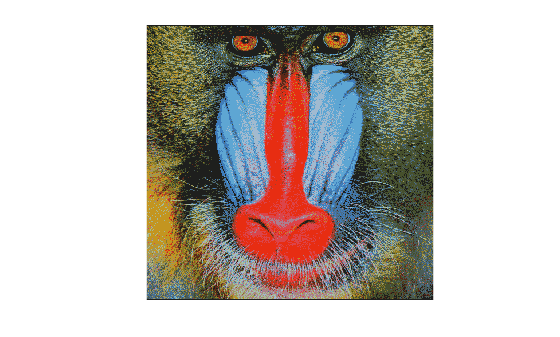Main Content

# imapprox

Approximate indexed image by reducing number of colors

## Syntax

``[Y,newmap] = imapprox(X,map,Q)``
``[Y,newmap] = imapprox(X,map,tol)``
``Y = imapprox(X,map,inmap)``
``___ = imapprox(___,dithering)``

## Description

example

````[Y,newmap] = imapprox(X,map,Q)` approximates the colors in indexed image `X` and associated colormap `map` by using minimum variance quantization with `Q` quantized colors. `imapprox` returns the indexed image `Y` with colormap `newmap`.```
````[Y,newmap] = imapprox(X,map,tol)` approximates the colors in indexed image `X` and associated colormap `map` by using uniform quantization with tolerance `tol`.```
````Y = imapprox(X,map,inmap)` approximates the colors in indexed image `X` and associated colormap `map` by using inverse colormap mapping with colormap `inmap`. The inverse colormap algorithm finds the colors in `inmap` that best match the colors in `map`.```
````___ = imapprox(___,dithering)` enables or disables dithering.```

## Examples

collapse all

Load an indexed image of a mandrill's face. Display image `X` using its associated colormap, `map`, which has 220 colors.

```figure load mandrill image(X) colormap(map) axis off axis image```Reduce the number of colors in the indexed image from 220 to only 16 colors by producing a new image, `Y`, and its associated colormap, `newmap`.

```figure [Y,newmap] = imapprox(X,map,16); image(Y) colormap(newmap) axis off axis image```## Input Arguments

collapse all

Indexed image with many colors, specified as an m-by-n matrix of nonnegative integers.

Data Types: `single` | `double` | `uint8` | `uint16`

Colormap with many colors associated with indexed image `X`, specified as a d-by-3 matrix with values in the range [0, 1]. Each row of `map` is a three-element RGB triplet that specifies the red, green, and blue components of a single color of the colormap.

Data Types: `double`

Number of quantized colors used for minimum variance quantization, specified as a positive integer that is less than or equal to 65,536. The returned colormap `newmap` has `Q` or fewer colors.

Tolerance used for uniform quantization, specified as a number in the range [0, 1]. The returned colormap `newmap` has `(floor(1/tol)+1)^3` or fewer colors.

Colormap with fewer colors used for inverse colormap mapping, specified as a c-by-3 matrix with values in the range [0, 1]. Each row of `inmap` is a three-element RGB triplet that specifies the red, green, and blue components of a single color of the colormap. The colormap has a maximum of 65,536 colors.

Data Types: `double`

Perform dithering, specified as `'dither'` or `'nodither'`. Dithering increases the color resolution at the expense of spatial resolution. For more information, see `dither`.

If you select `'nodither'`, then `imapprox` does not perform dithering. Instead, the function maps each color in the original image to the closest color in the new colormap.

## Output Arguments

collapse all

Indexed image with fewer colors, returned as an m-by-n matrix of positive integers. If the length of `newmap` (or `immap`, if specified) is less than or equal to 256, then the output image is of class `uint8`. Otherwise, the output image is of class `double`.

Data Types: `double` | `uint8`

Colormap with fewer colors associated with the output indexed image `Y`, returned as a c-by-3 matrix with values in the range [0, 1]. Each row of `newmap` is a three-element RGB triplet that specifies the red, green, and blue components of a single color of the colormap. The colormap has a maximum of 65,536 colors.

Data Types: `double`

## Algorithms

`imapprox` uses `rgb2ind` to create a new colormap that uses fewer colors. For more information about quantization and inverse colormap mapping, see the Algorithms of `rgb2ind`.

## See Also

### Topics

Introduced before R2006a

Download ebook